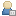###Show Posts

This section allows you to view all posts made by this member. Note that you can only see posts made in areas you currently have access to.

### Messages - MichaelPetty

Pages: 
1
##### Transformer calculator (Public) / Re: Measuring and calculating permeability of unknown cores
« on: September 01, 2019, 12:05:00 PM »
I have a lot of unknown ferrite cores mostly salvaged from radio/TV and other devices.
Main problem with this is to how to know their permeability for calculating number of turns I need to make choke or transformer.

So I needed some simple method to test cores and get theirs permeability.
Simplest way to me is to measure inductivity of choke with unknown core when you know how many turns of wire you have on it.
Then calculate from this inductance factor AL and effective permeability μe of core.

Formulas
Usually you can find formulas for calculating in manufacturer Data Handbook or production guide.
The AL factor is the inductance per turn squared (in nH) for a given core.
Inductance formula is: L = N2*AL (nH)
When transformed, you can calculate AL with next formula: AL=L/N2
Once you have core data, value of AL is calculated from the core factor Σ(l ⁄A) and the effective permeability: AL= (μ0e*106)/ Σ(l ⁄A) (nH)

To get effective permeability, formula is transformed into this: μe = (AL*Σ(l ⁄A))/(μ0*106)
AL is calculated from measured inductance and number of turns.
Σ(l ⁄A) is calculated by measuring and dividing core physical dimensions of Dbal review effective length le (mm) and effective area Ae (mm2): Σ(l ⁄A) = le/Ae (mm-1)
μ0 is permeability of vacuum - μ0 = 4*π*10-7 (Hm-1)

This formulae works for most core types except for rods and tubes, for them you need this formula: L = (μ0rod*N2*A)/l (in H)
After transforming we get: μrod = (L*l)/(μ0*N2*A)
where:
L is measured inductance
N is number of turns
A is cross sectional area of rod (mm2)
l is length of coil. (mm)

Testing
With this solved only thing to do is to determine optimal number of turns for measurement.
By using large number of turns there is problem with rise of loses by wire resistance, coil geometry and core loses.
Therefore best is to use fewer as possible.
With minimal number of turns, wire length is reduced, thus wire resistance is low.
Beside that with few turns we don't have overlapping wire and loses in coil construction.
However when using single or few turns there is problem with covering entire space on coil former to get fully distributed winding to use entire length of core.

So to solve that problem I did some testing. For my test I chose RM6S/I ferrite core.
I was using three different core materials 3H3, 3C90 and 3E5 and I make coils with different number of turns, form 1 to 31.
For measuring inductance I was using Agilent U1731C LCR meter (resolution 1-100nH, accuracy 1%) with 100 Hz and 1 kHz setup.

Wire thickness is another important part, if it is too thick you can get losses from skin effect.
On another hand if you use too thin wire you can get losses in wire resistance.
For my setup maximal measuring frequency is 1 kHz and on that frequency skin depth is 2.088 mm, thus wire must not be larger than AWG 6.
I choose to use SWG 25 (diameter 0.5 mm) with max frequency of about 80 kHz. Using this wire I can fit 9 turns on former in single row.

Measuring
One set of measuring was done from 1 to 31 turns with all cores exchanged on that turn. I repeat that set three times.
At end after several hundreds core swapping I compare readings from beginning and end.
Difference in data is from -4.62% to 43.37% which is primary result of loosening mounting clip after so many reassembling.
Most difference (higher than 10%) I got after 22nd turn which just confirms that less turns are far better for precision even in loose clip mounting.

Comparing 100 Hz vs. 1 kHz measurement data gave me difference from -7.51% to 0.98% which is not that bad.
Data from 1 kHz are much more close to datasheet values so it confirms that higher frequencies are better for measuring lower inductivity.

Data
All processed data are from measurement at 1 kHz setup. Those values are used to calculate AL and compared with value from datasheet.Here on this graph you can see difference for each core material.
For 3H3 material stable results is between 5th and 20th turn, for 3C90 is from 3rd and 31st turn and for 3E5 it looks there aren't stable results however after 21st turn difference are gone totally wild.
So it is safe to say that measuring from 5 to 20 turns gives best results.

Conclusion
To get and calculate permeability the best method is:
• if possible, use high measuring frequency but close to working frequency of core
• use 5 to 20 turns (closer to 5 turns for high amplification cores)
• use thickest wire possible depend on measuring frequency and space on former
• fill up equally entire length of former in single row

I was also feeling the same problem and when i did testing of the formulae i failed.
Thanks for the data. It was helpful to me and my friends.

Pages: